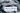# Leetcode - Split a String Into the Max Number of Unique Substrings

October 04, 2020## Problem Statement

Given a string s, return the maximum number of unique substrings that the given string can be split into.

You can split string s into any list of non-empty substrings, where the concatenation of the substrings forms the original string. However, you must split the substrings such that all of them are unique.

A substring is a contiguous sequence of characters within a string.

Example

``````Input: s = "ababccc"
Output: 5
Explanation: ['a', 'b', 'ab', 'c', 'cc']

Input: s = "aba"
Output: 2
Explanation: ['a', 'ba']
Example 3:

Input: s = "aa"
Output: 1
Explanation: It is impossible to split the string any further.``````

## Solution

Problems like these are solved through recursions, where you want to extract a portion of string and repeat the same algorithm on the rest of string.

Lets look at the algorithm:

• For checking unique substrings, you need to take a HashSet
• We would take a substring, check it if its unique.
• If its unique, we will move forward with rest of string in the same algorithm.

Lets look at one of example:

``````input=aba

a + (ba)
a + b + (a)
# a is duplicate, it will only (a, b)

Next iteration
ab + (a)

Next Iteration
aba + ()
``````

### Code

Lets look at the code:

``````private int find(String s, Set<String> set) {
int max = 0;
for (int i=0; i<s.length(); i++) {
String sub = s.substring(0, i+1);
if (!set.contains(sub)) {
max = Math.max(max, 1 + find(s.substring(i+1), set));

set.remove(sub);
}
}

return max;
}

public int maxUniqueSplit(String s) {
Set<String> set = new HashSet<>();
return this.find(s, set);
}``````

### Complexity

Its `O(n!)`, factorial of n.